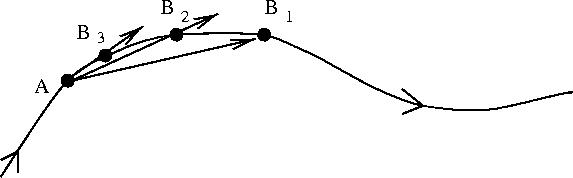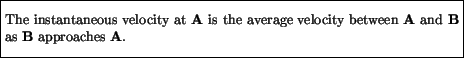Next: Acceleration Up: Motion Previous: Position

# Velocity

For an object in motion the concept of velocity is important. There are two types of velocity that can be defined. Suppose an object moves from one point to another in a certain time interval. The average velocity during that time interval is defined asNote that, as with the position, the velocity is a vector: it has a magnitude and a direction associated with it. For example, if someone travels from Toronto to Vancouver by airplane in three hours, their average velocity for that trip is 3000 km divided by 3 hours, or 1000 km/hr, due east. Sometimes only the magnitude of the velocity is of interest. It is called the speed. The average speed for the above trip from Toronto to Vancouver is 1000 km/hr. No direction need be specified. Note that objects moving with a large speed change their position quickly over time, whereas those moving with a small speed take longer to cover the same distance.

The average velocity only depends on the initial and final points of the travel - it knows nothing of the details of the motion between these points. For this reason one introduces the concept of instantaneous velocity, which applies to a single point, or time of the motion. The instantaneous velocity is defined as follows. Suppose one wants to define the instantaneous velocity at a certain point, say A along the journey. One first calculates the average velocity between this point and an arbitrary nearby point, say B1. The arrow in the Figure below pointing from A to B1 indicates the direction of the average velocity in going between those two points. One then does the same, but for a point B2 which is nearer to the initial point A of interest. Then again for B3 which is closer still, and so on. The instantaneous velocity is obtained by repeating this process until you get to a point B that is arbitrarily close to A.Thus the definition of instantaneous velocity is:As the Figure indicates, as the B gets closer and closer to A the direction of the velocity (indicated by the arrows) gets closer and closer to pointing along a line that is tangent to the trajectory at the point A (i.e. points along the trajectory). The instantaneous speed is correspondingly defined as the magnitude of the instantaneous velocity. An example with which we are all familiar involves travel by car. Suppose we are driving between Winnipeg and Kenora. This road is more or less completely straight, so we don't have to worry about directions. After two hours we stop for a one hour lunch break, and then continue to drive for another hour. At this stage, the odometer shows that we have driven 300 km, so that our average speed for that portion of the trip would be 300/4 = 75 km/hr. The magnitude of our instantaneous speed, on the other hand is given by the speedometer reading, and can be very different from the average speed. For example we might be going at 100 km/hr or if we happen to be stopped at a traffic light, our instantaneous speed might be zero. In effect, the odometer counts the total number of revolutions made by the tires (and multiplies by the circumference of the tire) in order to get the total distance traveled. The speedometer instead counts the number of revolutions in a very short time interval, say half a second, in order to tell us how fast the car is going at that instant.Next: Acceleration Up: Motion Previous: Position
modtech@theory.uwinnipeg.ca
1999-09-29